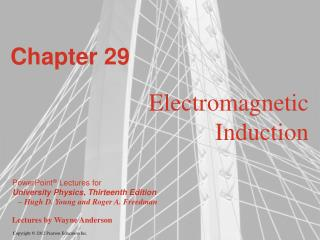DownloadDownload PresentationChapter 29

# Chapter 29

Download Presentation## Chapter 29

- - - - - - - - - - - - - - - - - - - - - - - - - - - E N D - - - - - - - - - - - - - - - - - - - - - - - - - - -
##### Presentation Transcript

1. Chapter 29 Electromagnetic Induction

2. Goals for Chapter 29 • To examine experimental evidence that a changing magnetic field induces an emf • To learn how Faraday’s law relates the induced emf to the change in flux • To determine the direction of an induced emf • To calculate the emf induced by a moving conductor • To learn how a changing magnetic flux generates an electric field • To study the four fundamental equations that describe electricity and magnetism

3. Introduction • How is a credit card reader related to magnetism? • Energy conversion makes use of electromagnetic induction. • Faraday’s law and Lenz’s law tell us about induced currents. • Maxwell’s equations describe the behavior of electric and magnetic fields in any situation.

4. Induced current • A changing magnetic flux causes an induced current. See Figure 29.1 below. • The induced emf is the corresponding emf causing the current.

5. Magnetic flux through an area element • Figure 29.3 below shows how to calculate the magnetic flux through an element of area.

6. Faraday’s law • The flux depends on the orientation of the surface with respect to the magnetic field. See Figure 29.4 below. • Faraday’s law: The induced emf in a closed loop equals the negative of the time rate of change of magnetic flux through the loop, or  = –dB/dt.

7. Emf and the current induced in a loop • Follow Example 29.1 using Figure 29.5 below.

8. Direction of the induced emf • Follow the text discussion on the direction of the induced emf, using Figure 29.6 below.

9. Magnitude and direction of an induced emf • Read Problem-Solving Strategy 29.1. • Follow Example 29.2 using Figure 29.7 below.

10. A simple alternator • Follow Example 29.3 using Figures 29.8 (below) and 29.9 (right).

11. DC generator and back emf in a motor • Follow Example 29.4 using Figure 29.10 below.

12. Slidewire generator • Follow Example 29.5 using Figure 29.11 below.

13. Work and power in the slidewire generator • Follow Example 29.6 using Figure 29.12 below.

14. Lenz’s law • Lenz’s law: The direction of any magnetic induction effect is such as to oppose the cause of the effect. • Follow Conceptual Example 29.7.

15. Lenz’s law and the direction of induced current • Follow Example 29.8 using Figures 29.13 (right) and 29.14 (below).

16. Motional electromotive force • The motional electromotive force across the ends of a rod moving perpendicular to a magnetic field is = vBL. Figure 29.15 below shows the direction of the induced current. • Follow the general form of motional emf in the text.

17. A slidewire generator and a dynamo • Follow Example 29.9 for the slidewire generator. • Follow Example 29.10 for the Faraday disk dynamo, using Figure 29.16 below.

18. Induced electric fields • Changing magnetic flux causes an induced electric field. • See Figure 29.17 at the right to see the induced electric field for a solenoid. • Follow the text discussion for Faraday’s law restated in terms of the induced electric field. • Follow Example 29.11 using Figure 29.17.

19. Eddy currents • Follow the text discussion of eddy currents, using Figure 29.19 at the right.

20. Using eddy currents • Figure 29.20 below illustrates an airport metal detector and a portable metal detector, both of which use eddy currents in their design.

21. Displacement current • Follow the text discussion displacement current using Figures 29.21 and 29.22 below.

22. Maxwell’s equations • Maxwell’s equations consist of • Gauss’s law for the electric field • Gauss’s law for the magnetic field • Ampere’s law • Faraday’s law. • Follow the text discussion for the mathematical form of these four fundamental laws.

23. Superconductivity • When a superconductor is cooled below its critical temperature, it loses all electrical resistance. • Follow the text discussion using Figures 29.23 (below) and 29.24 (right).